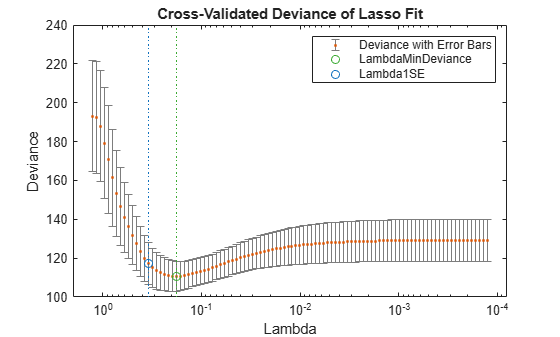Documentation

## Regularize Poisson Regression

This example shows how to identify and remove redundant predictors from a generalized linear model.

Create data with 20 predictors, and Poisson responses using just three of the predictors, plus a constant.

```rng('default') % for reproducibility X = randn(100,20); mu = exp(X(:,[5 10 15])*[.4;.2;.3] + 1); y = poissrnd(mu);```

Construct a cross-validated lasso regularization of a Poisson regression model of the data.

`[B,FitInfo] = lassoglm(X,y,'poisson','CV',10);`

Examine the cross-validation plot to see the effect of the `Lambda` regularization parameter.

```lassoPlot(B,FitInfo,'plottype','CV'); legend('show') % show legend```The green circle and dashed line locate the `Lambda` with minimal cross-validation error. The blue circle and dashed line locate the point with minimal cross-validation error plus one standard deviation.

Find the nonzero model coefficients corresponding to the two identified points.

`minpts = find(B(:,FitInfo.IndexMinDeviance))`
```minpts = 7×1 3 5 6 10 11 15 16 ```
`min1pts = find(B(:,FitInfo.Index1SE))`
```min1pts = 3×1 5 10 15 ```

The coefficients from the minimal plus one standard error point are exactly those coefficients used to create the data.

Find the values of the model coefficients at the minimal plus one standard error point.

`B(min1pts,FitInfo.Index1SE)`
```ans = 3×1 0.2903 0.0789 0.2081 ```

The values of the coefficients are, as expected, smaller than the original `[0.4,0.2,0.3]`. Lasso works by "shrinkage," which biases predictor coefficients toward zero.

The constant term is in the `FitInfo.Intercept` vector.

`FitInfo.Intercept(FitInfo.Index1SE)`
```ans = 1.0879 ```

The constant term is near 1, which is the value used to generate the data.